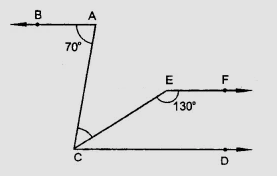"
">

# In the figure, if $AB \parallel CD$ and $CD \parallel EF$, find $\angle ACE$."

Given:

$AB \parallel CD$ and $CD \parallel EF$.

To do:

We have to find $\angle ACE$.

Solution:

We know that,

Vertically opposite angles are equal.

Corresponding angles are equal.

Alternate angles are equal.

Sum of the co-interior angles is $180^o$.

Therefore,

$\angle BAC = 70^o, \angle CEF = 130^o$

$\angle ECD + \angle CEF = 180^o$              (Co-interior angles)

$\angle ECD + 130^o = 180^o$

$\angle ECD = 180^o - 130^o$

$\angle ECD = 50^o$

$\angle BAC = \angle ACD$                           (Alternate angles)

$\angle ACD = 70^o$

$\angle ACE = \angle ACD - \angle ECD$

$\angle ACE = 70^o - 50^o$

$\angle ACE = 20^o$

Hence, $\angle ACE = 20^o$.

Updated on: 10-Oct-2022

45 Views